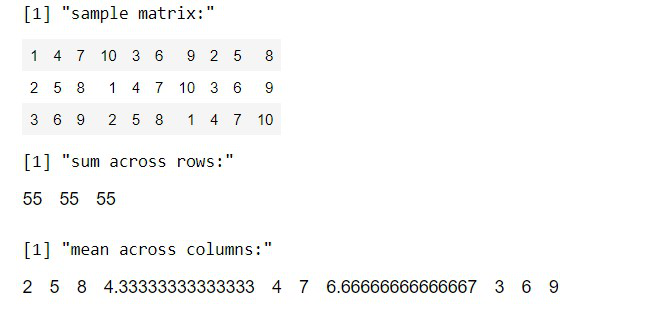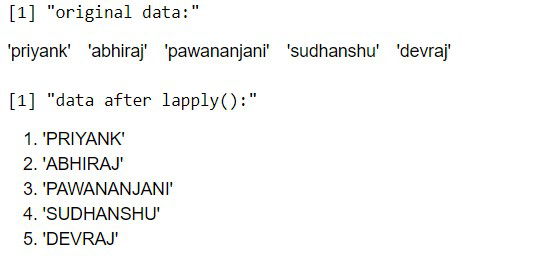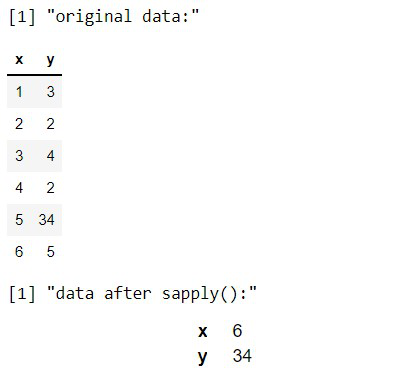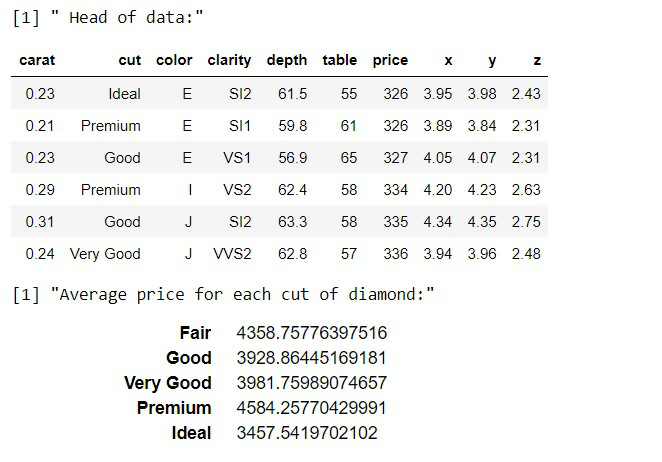GeeksforGeeks App
Open AppBrowser
Continue

# apply(), lapply(), sapply(), and tapply() in R

In this article, we will learn about the apply(), lapply(), sapply(), and tapply() functions in the R Programming Language.

The apply() collection is a part of R essential package. This family of functions helps us to apply a certain function to a certain data frame, list, or vector and return the result as a list or vector depending on the function we use. There are these following four types of function in apply() function family:

## apply() function

The apply() function lets us apply a function to the rows or columns of a matrix or data frame. This function takes matrix or data frame as an argument along with function and whether it has to be applied by row or column and returns the result in the form of a vector or array or list of values obtained.

Syntax: apply( x, margin, function )

Parameters:

• x: determines the input array including matrix.
• margin: If the margin is 1 function is applied across row, if the margin is 2 it is applied across the column.
• function: determines the function that is to be applied on input data.

Example:

Here, is a basic example showcasing the use of apply() function along rows as well as columns.

## R

 `# create sample data``sample_matrix <- ``matrix``(C<-(1:10),nrow=3, ncol=10)`` ` `print``( ``"sample matrix:"``)``sample_matrix`` ` `# Use apply() function across row to find sum``print``(``"sum across rows:"``)``apply``( sample_matrix, 1, sum)`` ` `# use apply() function across column to find mean``print``(``"mean across columns:"``)``apply``( sample_matrix, 2, mean)`

Output:## lapply() function

The lapply() function helps us in applying functions on list objects and returns a list object of the same length. The lapply() function in the R Language takes a list, vector, or data frame as input and gives output in the form of a list object. Since the lapply() function applies a certain operation to all the elements of the list it doesn’t need a MARGIN.

Syntax: lapply( x, fun )

Parameters:

• x: determines the input vector or an object.
• fun: determines the function that is to be applied to input data.

Example:

Here, is a basic example showcasing the use of the lapply() function to a vector.

## R

 `# create sample data``names <- ``c``(``"priyank"``, ``"abhiraj"``,``"pawananjani"``,``           ``"sudhanshu"``,``"devraj"``)``print``( ``"original data:"``)``names`` ` `# apply lapply() function``print``(``"data after lapply():"``)``lapply``(names, toupper)`

Output:## sapply() function

The sapply() function helps us in applying functions on a list, vector, or data frame and returns an array or matrix object of the same length. The sapply() function in the R Language takes a list, vector, or data frame as input and gives output in the form of an array or matrix object. Since the sapply() function applies a certain operation to all the elements of the object it doesn’t need a MARGIN. It is the same as lapply() with the only difference being the type of return object.

Syntax: sapply( x, fun )

Parameters:

• x: determines the input vector or an object.
• fun: determines the function that is to be applied to input data.

Example:

Here, is a basic example showcasing the use of the sapply() function to a vector.

## R

 `# create sample data``sample_data<- ``data.frame``( x=``c``(1,2,3,4,5,6),``                          ``y=``c``(3,2,4,2,34,5))``print``( ``"original data:"``)``sample_data`` ` `# apply sapply() function``print``(``"data after sapply():"``)``sapply``(sample_data, max)`

Output:## tapply() function

The tapply() helps us to compute statistical measures (mean, median, min, max, etc..) or a self-written function operation for each factor variable in a vector. It helps us to create a subset of a vector and then apply some functions to each of the subsets. For example, in an organization, if we have data of salary of employees and we want to find the mean salary for male and female, then we can use tapply() function with male and female as factor variable gender.

Syntax: tapply( x, index,  fun )

Parameters:

• x: determines the input vector or an object.
• index: determines the factor vector that helps us distinguish the data.
• fun: determines the function that is to be applied to input data.

Example:

Here, is a basic example showcasing the use of the tapply() function on the diamonds dataset which is provided by the tidyverse package library.

## R

 `# load library tidyverse``library``(tidyverse)`` ` `# print head of diamonds dataset``print``(``" Head of data:"``)``head``(diamonds)`` ` `# apply tapply function to get average price by cut``print``(``"Average price for each cut of diamond:"``)``tapply``(diamonds\$price, diamonds\$cut, mean)`

Output:My Personal Notes arrow_drop_up